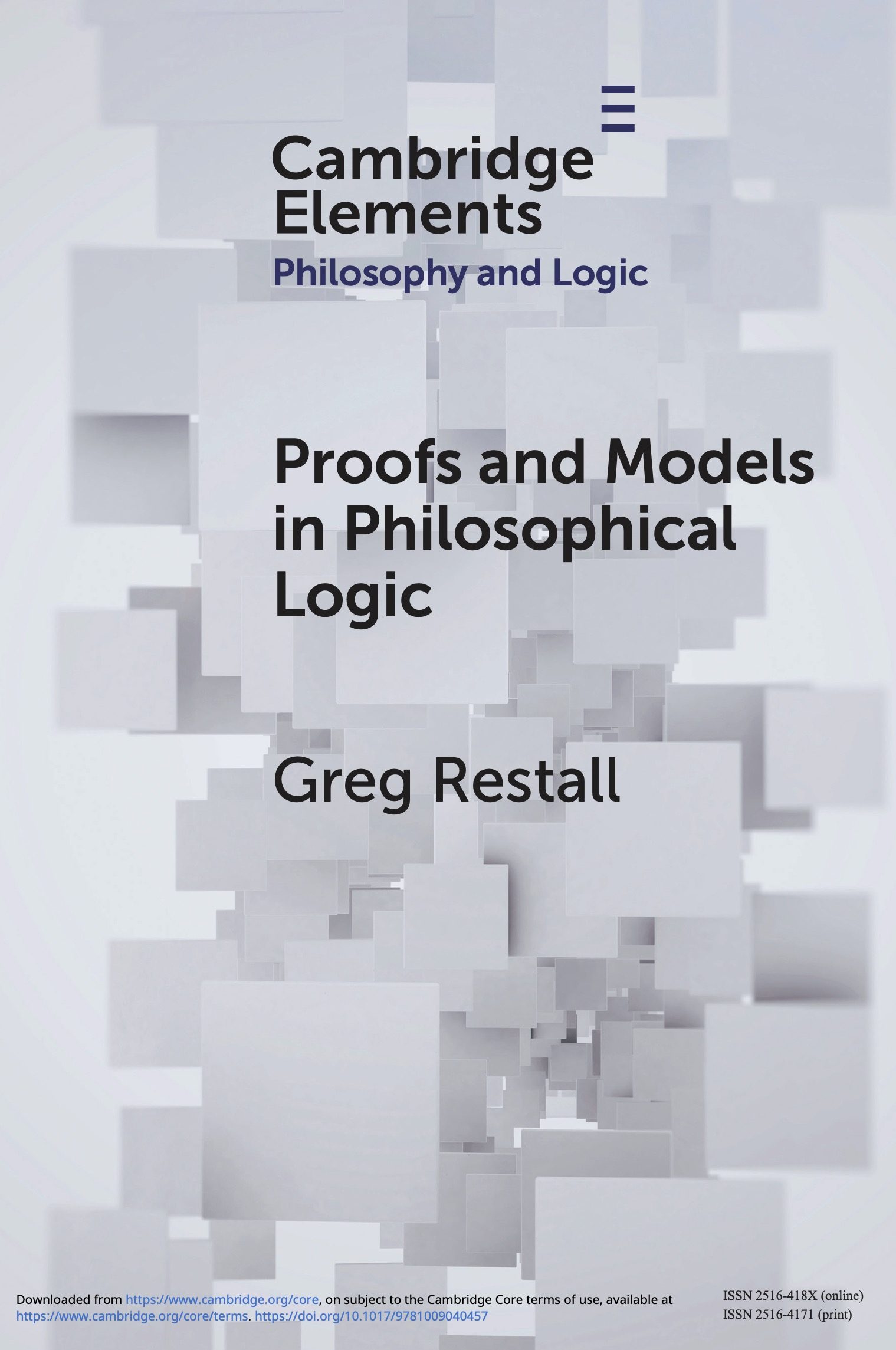# Proofs and Models in Philosophical Logic

## March 2022Proofs and Models in Philosophical Logic (Elements in Philosophy and Logic). Cambridge University Press, 2022.

This is a short book, in the Cambridge Elements series in Philosophical Logic.

This is a general introduction to recent work in proof theory and model theory of non-classical logics, with a focus on the application of non-classical logic to the semantic paradoxes and (to a lesser extent), the sorites paradox. After a short introduction motivating general notions of proof and of models, I introduce and motivate a simple natural deduction system, and present the structure of the liar paradoxical argument (concerning truth) and Curry’s paradox (concerning class membership). I introduce and motivate the notion of a structural rule, in both natural deduction and the sequent calculus and I compare and contrast the different approaches to substructural treatments of the paradox, contrasting the roles that contraction, cut and identity play in the derivations of the paradoxes.

In the next section, I introduce model theoretic treatments of the paradoxes, introducing supervaluations, and three-valued treatments of vagueness, and of the semantic paradoxes. I explain the fixed-point model construction that shows how to construct three-valued models for theories of truth, which can be used to then give models for different logics: K3 (with truth-value gaps), LP (with truth-value gluts) and ST (which supports all of classical logic, at the cost of invalidating the cut rule). I compare and contrast these approaches, and introduce the Routley—Meyer ternary relational semantics one way to model logics without the structural rules of contraction, or of weakening.

In the final section, I explore the relationship between proofs and models, explaining how the soundness and completeness theorems are proved for classical logic and for ST. I then discuss the issue of whether proofs or models play the primary semantic role, relating this question to the broader context of inferentialist and representationalist theories of meaning. This section closes with a discussion of the different resources that proof-first and model-first accounts of semantics have for answering the general question of how a language might be generally resistant to triviality due to paradox.

It is available from Cambridge University Press.